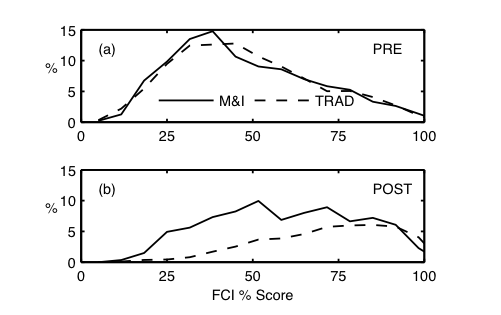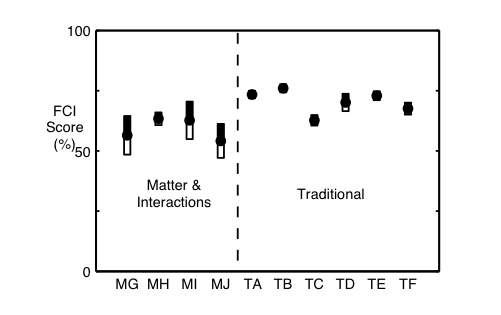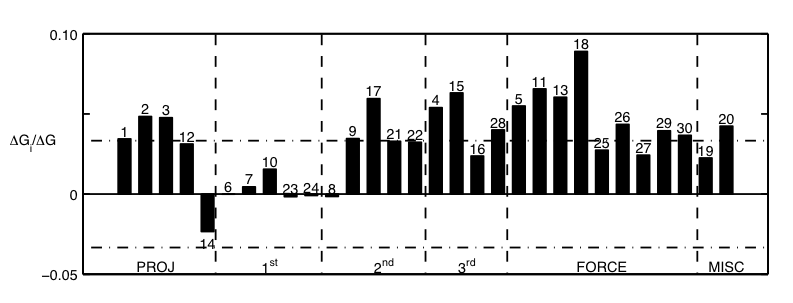Standardized Measures in Mechanics

The School of Physics has used the Force Concept Inventory (FCI) to assess the qualitative understanding gained by introductory mechanics students. The FCI is a 30-item multiple choice test focusing on the core concepts of force and motion. It does not cover other important mechanics topics including energy and angular momentum. The "distractors" (i.e., the incorrect answers) are very strong on this assessment, as the test was designed around naive notions of force and motion that introductory students have.

Students in the M&I course underperform on the FCI relative to their standard course (TRAD) cohorts. Work is in progress to investigate the reasons for this underperformance.Average pre- and post-instruction FCI scores at Georgia Tech - The average FCI post-test scores are shown for students who have completed a one-semester mechanics course with either the traditional (TRAD) or Matter & Interactions (M&I) curriculum. Additionally, the average FCI pre-test score are shown for students before instruction in either the TRAD or M&I curriculum. The number of students tested for each curriculum at each institution is indicated in the figure. The error bounds represent the 95% confidence intervals on the estimate of the average score.Gain in understanding of mechanics as measured by the FCI - The increase in student understanding resulting from a one-semester traditional (TRAD) or Matter & Interactions (M&I) course is measured using (a) the average raw gain G and (b) the average normalized gain g. The average FCI post-test scores are shown for students who have completed a one-semester mechanics course with either the traditional (TRAD) or Matter & Interactions (M&I) curriculum. The error bounds represent the 95% confidence intervals on the estimate of (a) the raw gain and (b) the normalized gain.Global FCI score distributions - (a) The distribution of FCI test scores for students before completing a mechanics course with either a traditional (dashed line) or M&I curriculum (solid line) are shown for data from GT. (b) The percentage of students with a given FCI test score is plotted for students who have completed a mechanics course with either a traditional (dashed line) or M&I curriculum (solid line) at GT. The total number of students tested in each curriculum at each institution is the same as in the first figure. The plots are constructed from binned data with bin widths equal to approximately 6.7% of the maximum possible FCI score (100%). The distributions appear sawtoothed because scores are discrete values.Average FCI scores by instructor - The average end-of-semester FCI scores for 4 M&I (MX) and 6 traditional (TX) sections are shown. The error bounds indicate 95% confidence intervals on the estimates of the average for each section. The number of students tested in a particular section is given by NO in the table below. All instructors used the Personal Response System (PRS) during lecture except for instructor TC.Fractional difference in performance for mechanics subtopics - The fractional difference in performance ΔGi/ΔG between traditional and M&I students at GT is shown for each question on the FCI. Positive (negative) ΔGi/ΔG indicates superior performance by traditional (M&I) students. The numerical labels indicate the corresponding question number in order of appearance on the FCI. The ΔGi/ΔG are grouped together into one of six topics: Projectile Motion (PROJ), Newton's first law (1st), Newton's second law (2nd), Newton's third law (3rd), Force Identification (FORCE), and Miscellaneous (MISC). The horizontal lines (dot-dash) correspond to ΔGi/ΔG = +/- 0.0333.Georgia Tech FCI test results are shown for twenty-two traditional sections (T1-T22) and six Matter & Interactions sections (M1-M6). Different lecturers are distinguished by a unique letter in column L. The average FCI score O for NO students completing the course is shown for all sections. Moreover, in those sections where data is available, the average FCI score I for NI students entering the course are indicated. Nm is the number of students in a given section who took the FCI both at the beginning and at the end of their mechanics course.

References

There are several references to consider when discussing the FCI. Those references are listed below:

1. Hestenes, D. and Wells, M. and Swackhamer, G., Force concept inventory, The Physics Teacher, vol. 30, pgs. 141-158, 1992
2. Huffman, D. and Heller, P., What does the Force Concept Inventory actually measure?, The Physics Teacher, vol. 33, pg 138, 1995
3. Hestenes, D. and Halloun, I., Interpreting the force concept inventory: A response to March 1995 critique by Huffman and Heller, The Physics Teacher, vol. 33, pg 502, 1995
4. Heller, P. and Huffman, D., Interpreting the force concept inventory: A reply to Hestenes and Halloun, The Physics Teacher, vol. 33, pg. 503, 1995
5. Henderson, C. and Heller, K. and Heller, P., Common concerns about the force concept inventory, The Physics Teacher, vol. 40, pgs. 542-542, 2002 [ link ]